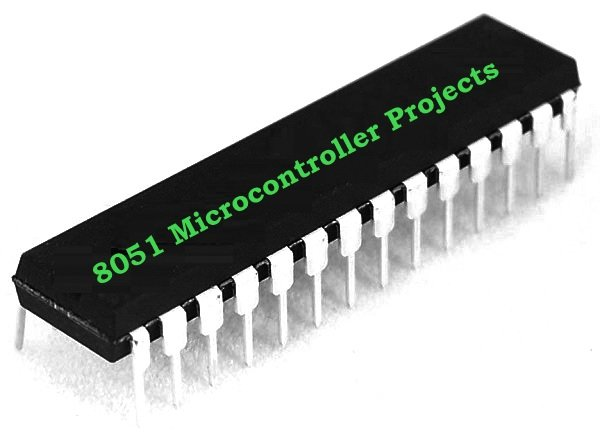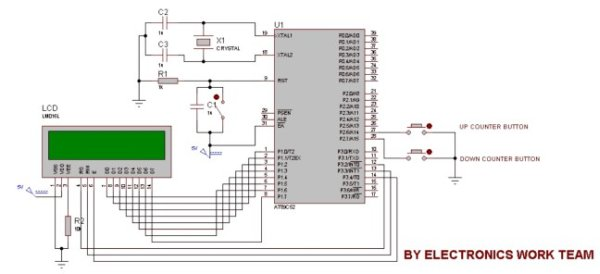# Up-Down counter on 16*2 LCD using 8051 microcontroller

DESCRIPTION

In this circuit 16*2 lcd IS used to show the value of count using 8051 microcontroller. The maximum value of count is 99 because. In this circuit we are using 8051-microcontroller, 16*2 lcd, 2 switches for up counting button & down counting button. Data pins of LCD are connected to P1 port of the 8051 microcontroller. UP counter button is connected with P2.6 and down counter button is connected with P2.7.Whenever the UP counter button is pressed the counter increments by one and when the down counter button is pressed it gets reduced by one.PROJECT CODE

#include< reg51.h >

sfr lcddata=0x90; //LCD DATA PINS
sbit rs=P3^2;
sbit rw=P3^3;
sbit en=P3^4;
sbit g=P2^6;
sbit h=P2^7;

int m=0;
int a,b;
unsigned char n={0x30,0x31,0x32,0x33,0x34,0x35,0x36,0x37,0x38,0x39};

void delay(unsigned char b)
{
unsigned char a;
for(b;b>0;b–)
{
for(a=500;a>0;a–);
}
}

void command(unsigned char dost)
{
lcddata=dost;
en=1;
rs=0;
rw=0;
delay(5);
en=0;
}

void lcddisplaydata(unsigned char n)
{
lcddata=n;
en=1;
rs=1;
rw=0;
delay(50);
en=0;
}

void main()
{
P2=0xFF;
command(0x38);
command(0x0C);
while(1)
{
if(g==0)
{
if(m==99)
{
command(0x80);
lcddisplaydata(n);
command(0x81);
lcddisplaydata(n);
}
else
{
m=m+1;
a=m/10;
{
command(0x80);
lcddisplaydata(n[a]);
}
b=m%10;
{
command(0x81);
lcddisplaydata(n[b]);
}
while(g==0);
}
}if(h==0)
{
if(m==0)
{
command(0x80);
lcddisplaydata(n);
command(0x81);
lcddisplaydata(n);
}
else
{
m=m-1;
a=m/10;
{
command(0x80);
lcddisplaydata(n[a]);
}
b=m%10;
{
command(0x81);
lcddisplaydata(n[b]);
}
while(h==0);
}
}
}
}

For more detail: Up-Down counter on 16*2 LCD using 8051 microcontroller

#### Current Project / Post can also be found using:

• up down counter using pic microcontroller
• up-down counter on 16×2 lcd using 8051 microcontroller
• pic16f84a lcd counter projects
• Counter frequency timer 0 lcd pic16f628a with button
READ  In circuit serial programmer for PIC16F87X